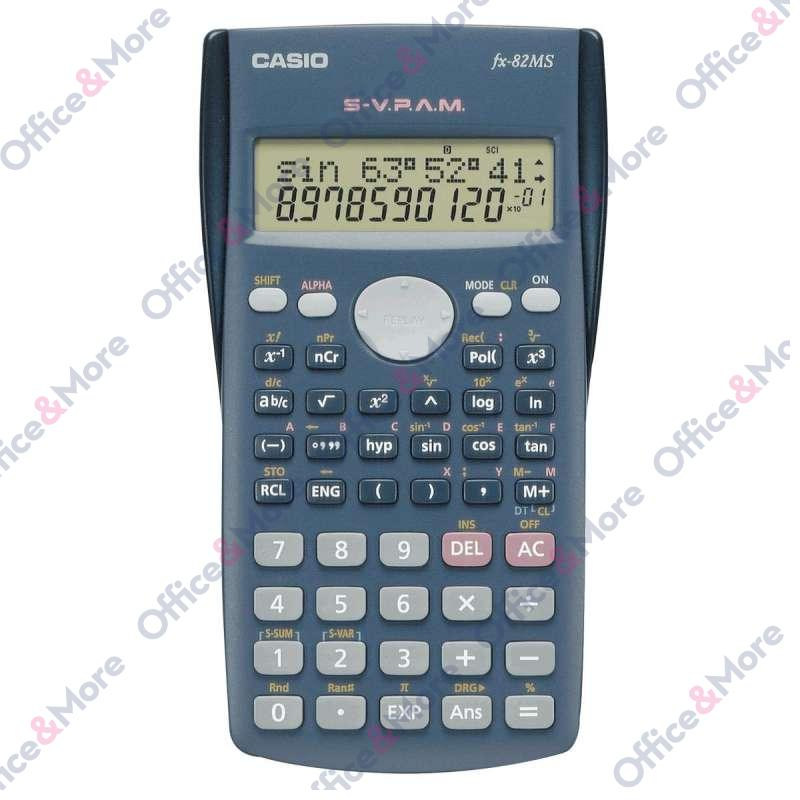CASIO

# CASIO KALKULATOR FX-82MS

Šifra artikla: 2501150103010
0,00 RSD
1.999,01 RSD
1.999,01 RSD
1.665,84 RSD
Ušteda: 0,00 RSD
Obavesti me o sniženju
Proveri dostupnost
Količina: 1 1
2
1
Sačuvajte u listi želja
Features overview

2-line display
12 and 10+2 digits, top line dot matrix, bottom line LCD
S-V.P.A.M. (algebraic input logic)
Trigonometric functions
8 variable memories
Two-dimensional statistics
Regression calculation
Repeat function
240 functions
Battery

Functions & general information
General information:

Scientific desktop-sized calculator
240 functions
Two-line display: the top line has a 12-character dot matrix display, the bottom line has an LCD 10 + 2 character display
Power supply: auxiliary battery 1 x AA
with a hard case
Dimensions (H x W x D): 18.6 x 85 x 156 mm
Weight: 125 g

Functions:

large cursor key for scrolling, recalling and editing calculations
clear display that includes a thousand separator, exponential display as a x10 symbol, the top line in a larger font size etc.
very convenient menu operation for statistical calculations
S-V.P.A.M.
1 independent memory
8 constant memories
SCI/FIX/ENG function
calculates percentages
24 levels of brackets
Trigonometric functions
Converts between polar coordinates and rectangular coordinates
hyperbolic and inverse hyperbolic functions
Converts between sexagesimal and decimal
calculates with fractions
Random number generator
two-dimensional statistics that calculates the average value, the estimated value and the total
permutation, combinational logic
calculates 6 different regression types and the coefficients A, B

Specs are subject to change w/o notice.
Karakteristika Vrednost
Kategorija Naučni kalkulatori
Robna Marka CASIO

### Ostavi komentar

Trenutno nema komentara